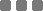## Saturday, October 6, 2018

### Math: Area of Equilateral Triangle (With Given Side Length)

The final form is \$A = {a²√3}/4\$

A is area.

a is the known side length.

Heron's formula

The formulation for arbitrary triangle, Heron's formula, is \$A = √{s(s-a)(s-b)(s-c)}\$

With (should) \$a ≥ b ≥ c\$

Where \$s = (a + b + c)/2\$

a, b, and c are the lengths of each side.

The \$1/2\$ in \$s\$ is related to the center point (centroid) of the triangle.

Derivation

The equilateral triangle area formulation (final form above) is derived from Heron's formula. As in a = b = c, plug that into Heron's formula, from s then plug that to A, you will get same final (simplified) form.

So then, \$a = b = c\$. We just use one letter, \$a\$.

Substitute \$b\$ and \$c\$ as \$a\$ in \$s\$. Therefore, \$s = {(a + a + a)/2} = {3/2}a\$.

Plug the \$s\$ to \$A\$, also substitute \$b\$ and \$c\$ as \$a\$ in \$A\$.

So then, \$A = √{{3/2}a({3/2}a-a)({3/2}a-a)({3/2}a-a)}\$

Continue:

\$A = √{{3/2}a({1/2}a)({1/2}a)({1/2}a)}\$

\$A = √{{3/2}a{1/8}a³}\$

\$A = √{{3/16}a⁴}\$

\$A = √3√{1/16}√a⁴\$

\$A = √3{1/4}a²\$

\$A = {a²√3}/4\$

Basic: area of a triangle with base and height lengths

Anyhow, area of a triangle with known base and height is \$ A = {b×h}/2\$

b is base

h is height

The \$1/2\$ (divided by two or multiplied by half) is based on a diagonally and evenly cut rectangle or square, from one corner to the the other diagonally opposite corner into two pieces. Each piece is called a (right) triangle. You see, the area of rectangle is length times width, um and so on.

Why is it the area of rectangle length times width? Like this, let's visualise it using a foot or a stick with the same length and width, I mean we use bunch of feet or sticks with exactly the same size. It's about how many feet or sticks the rectangular-shaped area fully contains (without stacking them, because we're talking two dimensions). Thus, length times width.
Wait a second, doesn't foot have curves? Why a stick, not a small board?

Is there wrong triangle? Yes, there is. It's two-dimensional figure except triangle.

Back to Heron's

Heron's can be proven via law of cosines, or Pythagorean, or law of cotangents, or other which can be used to prove it.

How did Heron get to that? Well, actually, prior Heron there were also people who wrote stuffs for some reason. Who was the first to discover it? I don't know.

Heron's formula forms (area of a triangle with known all sides)

Base form is \$A = √{s(s-a)(s-b)(s-c)}\$

If we do not want to "abbreviate" the \${a + b + c}/2\$ as \$s\$, we'll find other equivalent forms, such as:

• \$A = {√{(a + b + c)(-a + b + c)(a - b + c)(a + b - c)}}/4\$
• \$A = {√{2(a²b² + a²c² + b²c²) - (a⁴ + b⁴ + c⁴)}}/4\$
• \$A = {√{(a² + b² + c²)² - 2(a⁴ + b⁴ + c⁴)}}/4\$
• \$A = {√{4(a²b² + a²c² + b²c²) - (a² + b² + c²)²}}/4\$

The [something]

...Math: Area of Equilateral Triangle (With Given Side Length)
https://monkeyraptor.johanpaul.net/2018/10/math-area-of-equilateral-triangle-with.html